05 Oct 2023

Posted on:

04 Oct 2023

0

# Resolved:Where did the instructor get the Probability Function for the binomial distribution at 4:08?

Where did the instructor get the Probability Function for the binomial distribution at 4:08?

i've also never seen the probability being raised to a power, what's the intuition/reason behind that?

thanks

Instructor
Posted on:

05 Oct 2023

1

Hey Ryan,

Thanks for reaching out!

Let's recall how the formula goes: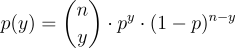The probability we are searching for, p(y), corresponds to the probability of obtaining y successes out of n trials. The first term in the equation, n-choose-y, is the number of ways in which we can obtain these successes. The second term, p to the power of y, is the probability of success multiplied by the number of successes we want at the end of the experiment. Analogously, the last term represents the probability of failure multiplied by the number of failures we want at the end of the experiment.

As an example, consider an experiment consisting of throwing a fair die three times. Throwing a six is considered a success. At the end of the experiment we want to have thrown exactly two sixes. What is the probability of this outcome?

First, we want to know in how many ways we can throw two sixes out of three rolls. The possibilities are the following:

- 66X

- 6X6

- X66

where an X denotes any throw different from a six. We can see that there are three ways in which we can do that. This is precisely the combination of choosing two elements out of three: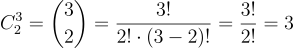Next, we need to multiply this number by the the probability of succeeding twice: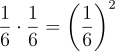And finally, we need to multiply the result by the probability of failing once: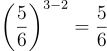The probability of obtaining a six two times out of three rolls is therefore: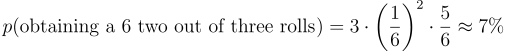Hope this example helps understanding the binomial distribution better.

Kind regards,

365 Hristina